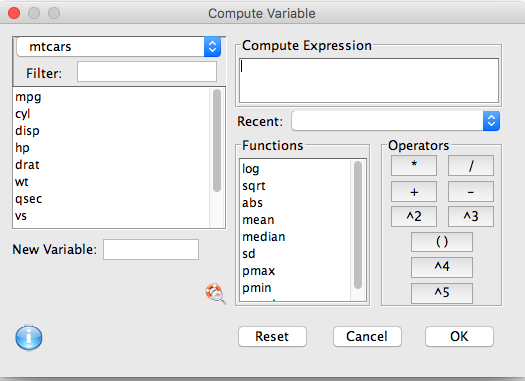Use the compute dialog to create new variables from existing ones using a formula.

The compute expression can take any valid R formula. For example you can make a new variable representing the sum of a number of existing variables.• ''Compute expression:' Build the desired compute expression here.
• Variable list: double click on any variable to insert it into the expression
• Operators: A series of common operators. x * y multiplies variables, x + y adds them, x - y subtracts, x / y divides, x^2 x^3 x^4 x^5 raises a variable to a power, and ( ) nests an expression (for example (x + y)^2).
• Functions: These are common functions for use in creating expressions. Select one and click the magnifying glass to open a help page for the function.
• New Variable: The name of the new variable to be created.